# How to Count Cells that do not Contain Errors in Excel

This post will guide you how to count the number of cells that do not contain errors within a range of cells using a formula in Excel 2013/2016 or Excel office 365. How do I count the number of cells without any errors using a simple formula in Excel.

## Count Number of Cells that do Not Contain Errors

You can use the SUMPRODUCT function in combining with NOT and ISERROR functions to count cells in a specified range of cells that do not contain errors

The below is a generic formula to count the number of cells that do not contain errors:

=SUMPRODUCT(–NOT(ISERR(range)))

Note: range is the range in which you want to count cells with no errors in the above generic form of the formula.

Supposing that you wish to count the number of cells in range B1:B6 that do not contain errors, and you can use the following formula:

=SUMPRODUCT(–NOT(ISERR(B1:B6)))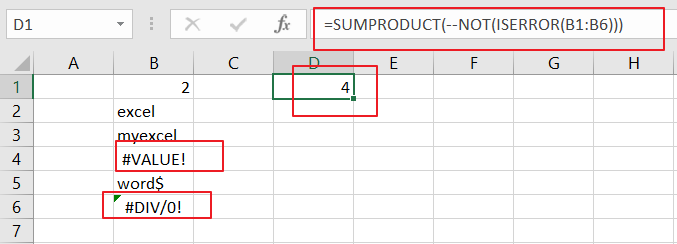LET’S SEE THAT HOW THIS FORMULA WORKS:

= NOT(ISERR(B1:B6))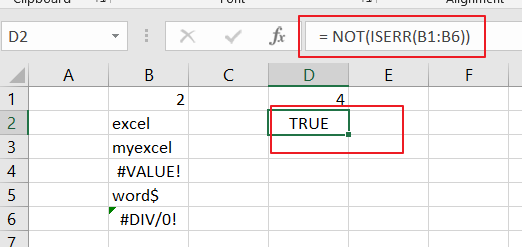The NOT and ISERROR functions can be used to identify the cells that do not contain an error. And it returns an array result with TRUE or FALSE values. The TRUE values indicate cells without errors, and FALSE values indicate cells with error values.

The array result is like this:

{TRUE;TRUE;TRUE;FALSE;TRUE;FALSE}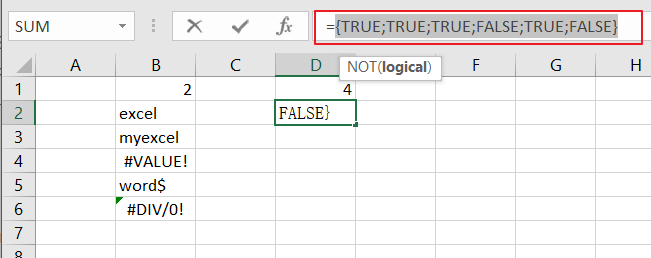= –NOT(ISERR(B1:B6))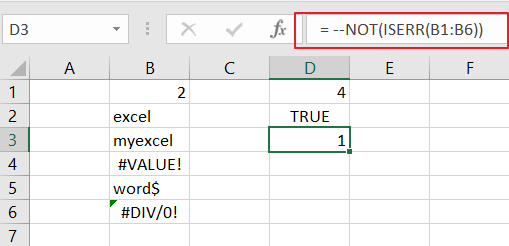Then you still need to convert the above array result to their numeric values, 1 and 0, so that the SUMPRODUCT function can calculate the sum of products of corresponding numbers. And you can do this with a double negative operator. And the final array result is like this:

{1;1;1;0;1;0}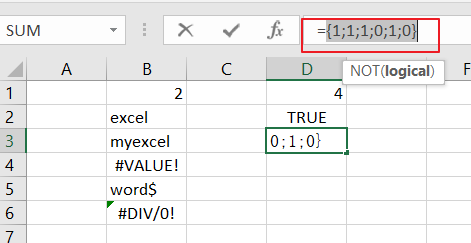The SUMPRODUCT function sums the items in the above array and return the final result.

You can also use the SUM function to count the cell that do not contain errors in range B1:B6. And the generic formula is similar with the above formula. It need to be entered as an array formula through pressing `CTRL +SHIFT + Enter` to convert it as array formula. The formula looks like this:

{=SUM(–NOT(ISERROR(B1:B6)))}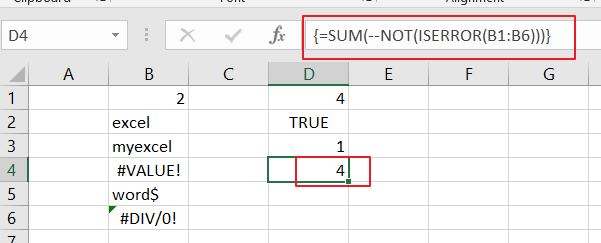### Related Functions

• Excel SUMPRODUCT function
The Excel SUMPRODUCT function multiplies corresponding components in the given one or more arrays or ranges, and returns the sum of those products. The syntax of the SUMPRODUCT function is as below:= SUMPRODUCT (array1,[array2],…)…
• Excel ISERROR function
The Excel ISERROR function used to check for any error type that excel generates and it returns TRUE for any error type, and the ISERR function also can be checked for error values except #N/A error, it returns TRUE while the error is #N/A. The syntax of the ISERROR function is as below:= ISERROR (value)….
• Excel SUM function
The Excel SUM function will adds all numbers in a range of cells and returns the sum of these values. You can add individual values, cell references or ranges in excel.The syntax of the SUM function is as below:= SUM(number1,[number2],…)…
• Excel NOT function
The Excel NOT function returns the opposite of a given logical or Boolean value. For example, if you supplied with the value TRUE, the NOT function will return FALSE; If you supplied with the value FALSE, and the NOT function will TRUE. The syntax of the NOT function is as below:=NOT(logical)…

Sidebar